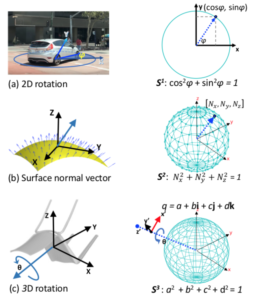# Spherical Regression: Learning Viewpoints, Surface Normals and 3D Rotations on n-SpheresThe CVPR 2019 paper Spherical Regression: Learning Viewpoints, Surface Normals and 3D Rotations on n-Spheres by Shuai Liao, Stratis Gavves and Cees Snoek is now available. Many computer vision challenges require continuous outputs, but tend to be solved by discrete classification. The reason is classification’s natural containment within a probability n-simplex, as defined by the popular softmax activation function. Regular regression lacks such a closed geometry, leading to unstable training and convergence to suboptimal local minima. Starting from this insight we revisit regression in convolutional neural networks. We observe many continuous output problems in computer vision are naturally contained in closed geometrical manifolds, like the Euler angles in viewpoint estimation or the normals in surface normal estimation. A natural framework for posing such continuous output problems are n-spheres, which are naturally closed geometric manifolds defined in the R^{(n+1)} space. By introducing a spherical exponential mapping on n-spheres at the regression output, we obtain well-behaved gradients, leading to stable training. We show how our spherical regression can be utilized for several computer vision challenges, specifically viewpoint estimation, surface normal estimation and 3D rotation estimation. For all these problems our experiments demonstrate the benefit of spherical regression. All paper resources are available at https://github.com/leoshine/Spherical_Regression.

This entry was posted in Uncategorized. Bookmark the permalink.